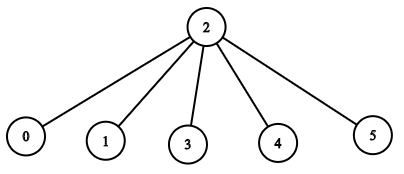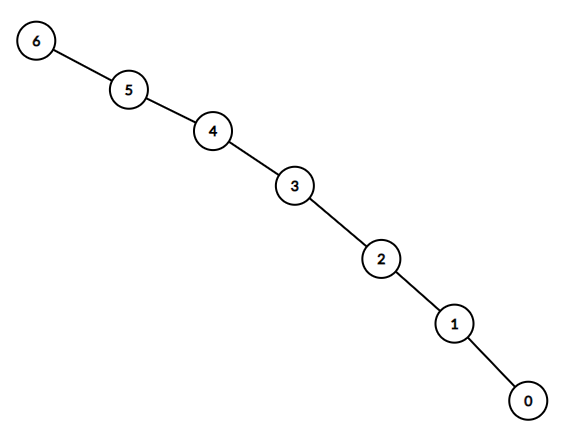Leetcode 1376：通知所有员工所需的时间（超详细的解法！！！）
in leetcode with 0 comment

manager 数组中，每个员工都有一个直属负责人，其中 manager[i] 是第 i 名员工的直属负责人。对于总负责人，manager[headID] = -1。题目保证从属关系可以用树结构显示。

i 名员工需要 informTime[i] 分钟来通知它的所有直属下属（也就是说在 informTime[i] 分钟后，他的所有直属下属都可以开始传播这一消息）。

输入：n = 1, headID = 0, manager = [-1], informTime =输入：n = 6, headID = 2, manager = [2,2,-1,2,2,2], informTime = [0,0,1,0,0,0]输入：n = 7, headID = 6, manager = [1,2,3,4,5,6,-1], informTime = [0,6,5,4,3,2,1]

id = 5 的员工将在 2 分钟内通知 id = 4 的员工。
id = 4 的员工将在 3 分钟内通知 id = 3 的员工。
id = 3 的员工将在 4 分钟内通知 id = 2 的员工。
id = 2 的员工将在 5 分钟内通知 id = 1 的员工。
id = 1 的员工将在 6 分钟内通知 id = 0 的员工。

输入：n = 15, headID = 0, manager = [-1,0,0,1,1,2,2,3,3,4,4,5,5,6,6], informTime = [1,1,1,1,1,1,1,0,0,0,0,0,0,0,0]

输入：n = 4, headID = 2, manager = [3,3,-1,2], informTime = [0,0,162,914]

• 1 <= n <= 10^5
• 0 <= headID < n
• manager.length == n
• 0 <= manager[i] < n
• manager[headID] == -1
• informTime.length == n
• 0 <= informTime[i] <= 1000
• 如果员工 i 没有下属，informTime[i] == 0
• 题目 保证 所有员工都可以收到通知。

• $f(father)=max(f(son))+informTime[t]$
class Solution:
def numOfMinutes(self, n: int, headID: int, manager: List[int], informTime: List[int]) -> int:
son = collections.defaultdict(list)
for i, v in enumerate(manager):
if v == -1: continue
son[v].append(i)

def dfs(x):
res = 0
for t in son[x]:
res = max(res, dfs(t))
return res + informTime[x]

return dfs(headID)

class Solution:
def numOfMinutes(self, n: int, headID: int, manager: List[int], informTime: List[int]) -> int:
son = collections.defaultdict(list)
for i, v in enumerate(manager):
if v == -1: continue
son[v].append(i)

def dfs(x):
return max([dfs(t) for t in son[x]] or ) + informTime[x]
return dfs(headID)

「如果我的文章对你有很大帮助，那么不妨～！」Responses